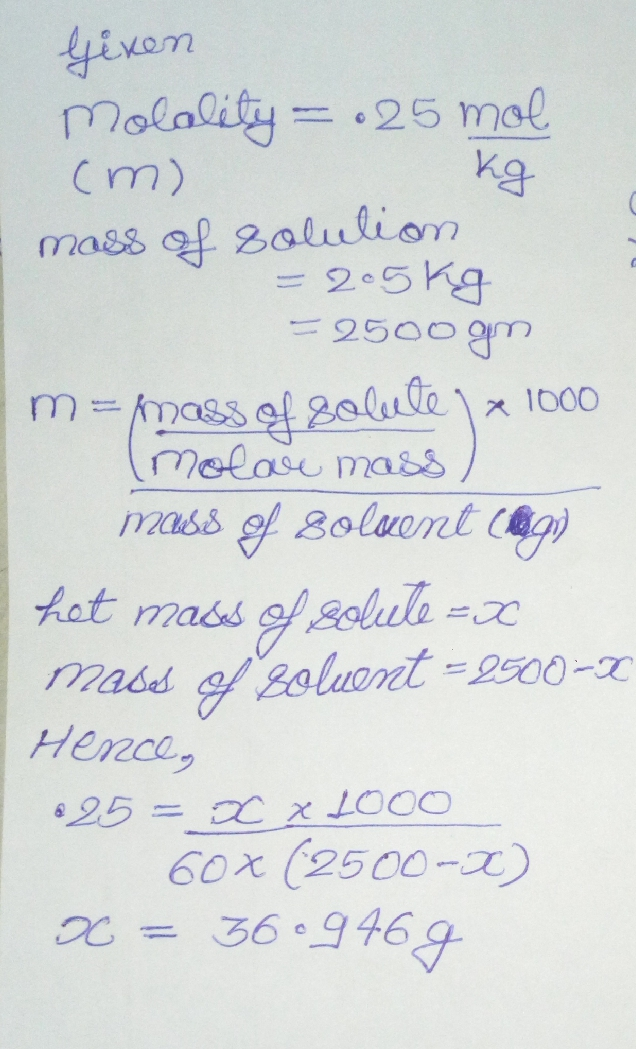×#### Thank you for registering.

One of our academic counsellors will contact you within 1 working day.

Click to Chat

1800-1023-196

+91-120-4616500

CART 0

• 0

MY CART (5)

Use Coupon: CART20 and get 20% off on all online Study Material

ITEM
DETAILS
MRP
DISCOUNT
FINAL PRICE
Total Price: Rs.

There are no items in this cart.
Continue Shopping
```
Calculate the mass of urea (NH2CONH2) required in making 2.5 kg of 0.25 molal aqueous solution.

```
8 years ago

```							Molar mass of urea (NH2CONH2) = 2(1 × 14 + 2 × 1) + 1 × 12 + 1 × 16 = 60 g mol−1
0.25 molar aqueous solution of urea means:
1000 g of water contains 0.25 mol = (0.25 × 60)g of urea = 15 g of urea i.e,
(1000 + 15) g of solution contains 15 g of urea
15×2500/1000+15 g

Therefore, 2.5 kg (2500 g) of solution contains  = 15×2500/1000+15 = 36.946 g = 37 g of urea (approximately) Hence, mass of urea required = 37 g
```
8 years ago
```							Let mass of urea = w gram Molar mass of urea = 60 gramMoles of urea = w/60 Weight of urea in kg= w/1000Molality =moles of solute/ mass of solvent  in kg0.25=(w/60)/{2.5- (w/1000)}w=36.9456 gram
```
2 years ago
```							Given,Molality(m) = 0.25 mol kg-1mass of the solution = 2.5 kg = 2500 gTo find,mass of urea required = ? [Molality = given mass of solute/molar mass × 1000/mass of the solvent (in g)] molar mass=(14+2+12+16+14+2) =60 g mol-1 Let x be the mass of urea required.mass of the solvent = (2500-x)substituting the values,0.25=x/60 ×1000/(2500-x)(2500-x)=x/60 ×1000/0.25(2500-x)=200x/32500=203x/3x=7500/203x=36.946 g
```
2 years ago
```							molality=number of moles of solute/mass of solvent in kg0.25=moles of solute/2.5moles of solute=0.25*2.5                        =0.625molar mass of urea(NH2CONH2)=14+2*1+12+16+14+2*1                                                      =60gmass of urea=0.625*60=37.5
```
one year ago
```							Hello students,The solution of the above problem is in the attached file.I hope the solution will solve all your doubts.Thank You,All the Best for the Exams.```
7 months ago
Think You Can Provide A Better Answer ?

## Other Related Questions on Organic Chemistry

View all Questions »### Course Features

• 731 Video Lectures
• Revision Notes
• Previous Year Papers
• Mind Map
• Study Planner
• NCERT Solutions
• Discussion Forum
• Test paper with Video Solution### Course Features

• 70 Video Lectures
• Revision Notes
• Test paper with Video Solution
• Mind Map
• Study Planner
• NCERT Solutions
• Discussion Forum
• Previous Year Exam Questions